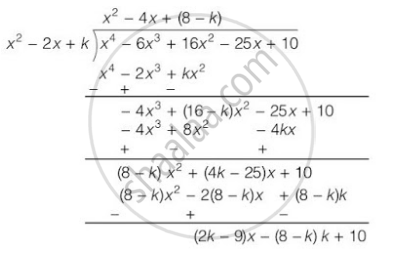# If the polynomial x^4 – 6x^3 + 16x^2 – 25x + 10 is divided by another polynomial x^2 – 2x + k, the remainder comes out to be x + a, find k and a. - Mathematics

If the polynomial x4 – 6x3 + 16x2 – 25x + 10 is divided by another polynomial x2 – 2x + k, the remainder comes out to be x + a, find k and a.

#### Solution

On dividing x4 – 6x3 + 16x2 – 25x + 10 by x2 – 2x + k∴ Remainder = (2k - 9)x - (8 - k)k + 10

But the remainder is given as x+ a.

On comparing their coefficients,

2k - 9 = 1

⇒ k = 10

⇒ k = 5 and,

-(8-k)k + 10 = a

⇒ a = -(8 - 5)5 + 10 =- 15 + 10 = -5

Hence, k = 5 and a = -5

Concept: Division Algorithm for Polynomials
Is there an error in this question or solution?
Chapter 2: Polynomials - Exercise 2.4 [Page 37]

#### APPEARS IN

NCERT Class 10 Maths
Chapter 2 Polynomials
Exercise 2.4 | Q 5 | Page 37

Share